## Partition Function Q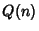gives the number of ways of writing the Integeras a sum of Positive Integers without regard to order with the constraint that all Integers in each sum are distinct. The values for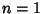, 2, ... are 1, 1, 2, 2, 3, 4, 5, 6, 8, 10, ... (Sloane's A000009). The Generating Function foris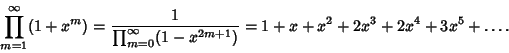The values offor whichis Prime are 3, 4, 5, 7, 22, 70, 100, 495, 1247, 2072, ... (Sloane's A046065), with no others for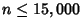.

The number of Partitions ofwith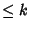summands is denoted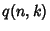or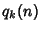. Therefore,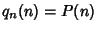and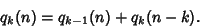Abramowitz, M. and Stegun, C. A. (Eds.). Partitions into Distinct Parts.'' §24.2.2 in Handbook of Mathematical Functions with Formulas, Graphs, and Mathematical Tables, 9th printing. New York: Dover, pp. 823-824, 1972.
Sloane, N. J. A. Sequences A046065 and A000009/M0281 in An On-Line Version of the Encyclopedia of Integer Sequences.'' http://www.research.att.com/~njas/sequences/eisonline.html and Sloane, N. J. A. and Plouffe, S. The Encyclopedia of Integer Sequences. San Diego: Academic Press, 1995.